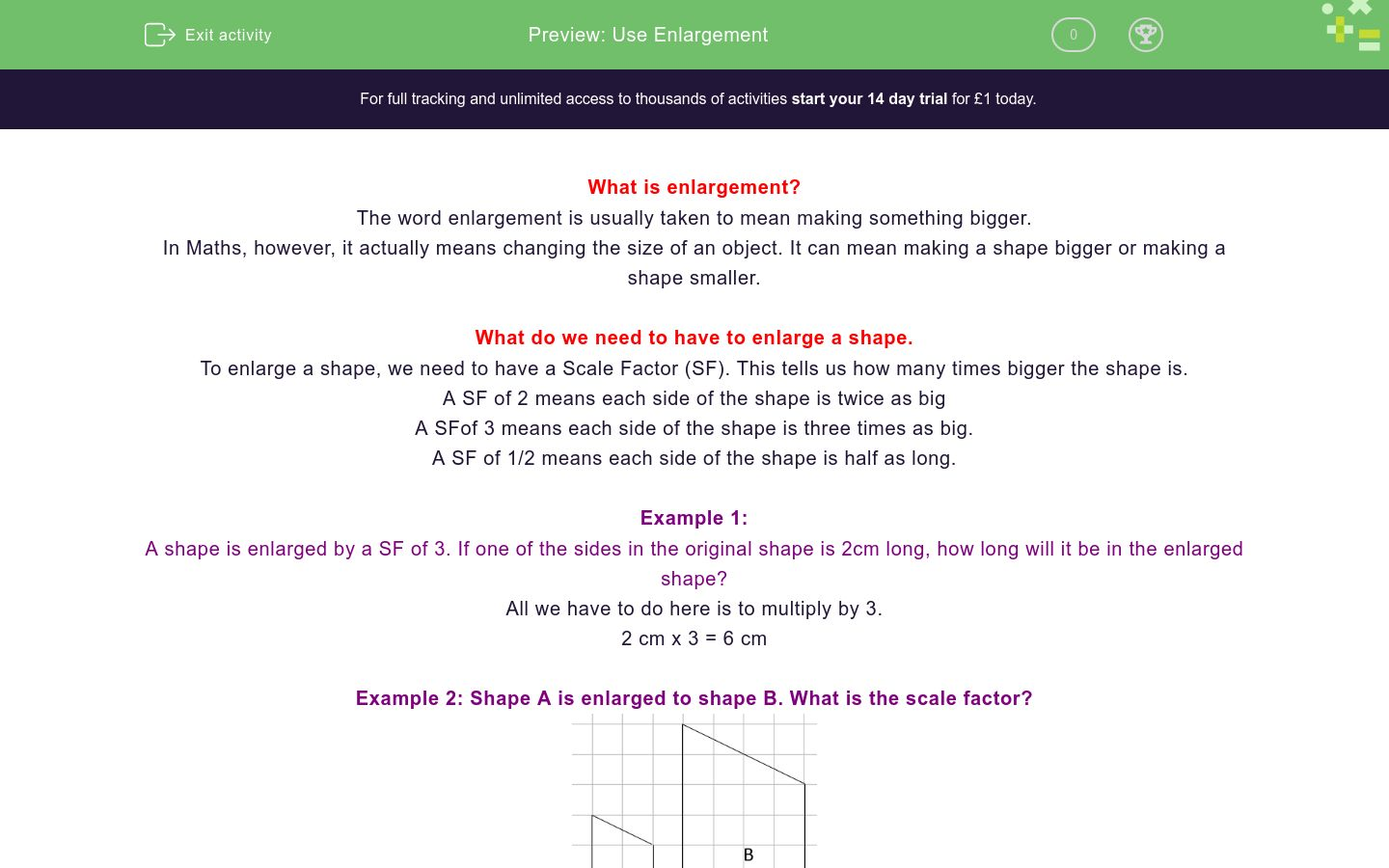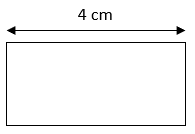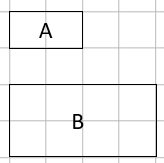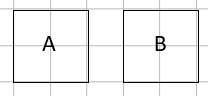# Use Enlargement

In this worksheet, students practise enlarging shapes.Key stage:  KS 4

GCSE Subjects:   Maths

GCSE Boards:   AQA, Eduqas, Pearson Edexcel, OCR

Curriculum topic:   Geometry and Measures, Congruence and Similarity

Curriculum subtopic:   Properties and Constructions, Plane Isometric Transformations

Difficulty level:### QUESTION 1 of 10

What is enlargement?

The word enlargement is usually taken to mean making something bigger.

In Maths, however, it actually means changing the size of an object. It can mean making a shape bigger or making a shape smaller.

What do we need to have to enlarge a shape.

To enlarge a shape, we need to have a Scale Factor (SF). This tells us how many times bigger the shape is.

A SF of 2 means each side of the shape is twice as big

A SFof 3 means each side of the shape is three times as big.

A SF of 1/2 means each side of the shape is half as long.

Example 1:

A shape is enlarged by a SF of 3. If one of the sides in the original shape is 2cm long, how long will it be in the enlarged shape?

All we have to do here is to multiply by 3.

2 cm x 3 = 6 cm

Example 2: Shape A is enlarged to shape B. What is the scale factor?Firstly, we need to say if the enlarged shape is bigger or smaller. As it is bigger, the SF will be a number greater than 1.

All we have to do here is to look at a pair of sides, it doesn't matter which ones as they will all give the same answer.

If we look at the left hand line in each shape, it is 3cm in shape A and 6cm in shape B. This means the SF = 2

Example 3: Shape B is enlarged to shape A. What is the scale factor?Firstly, we need to say if the enlarged shape is bigger or smaller. As it is smaller,  the SF will be a number between 0 and 1.

All we have to do here is to look at a pair of sides, it doesn't matter which ones as they will all give the same answer.

If we look at the left hand line in each shape, it is 3cm in shape A and 6cm in shape B. This means the SF = 1/2

Enlargement is where we make a shape...

To describe an enlargement we need a...

This shape is enlarged by a scale factor if 4.

How long will the marked side be in the enlarged shape?This shape is enlarged by a scale factor if 1/2.

How long will the marked side be in the enlarged shape?Shape A is enlarged to shape B. What is the Scale Factor?2

3

4

5

Shape B is enlarged to shape A. What is the Scale Factor?1/2

2

1/4

4

Shape A is enlarged to shape B. What is the Scale Factor?Shape B is enlarged to shape A. What is the Scale Factor?Shape A is enlarged to shape B. What is the Scale Factor?Shape A is enlarged to shape B. What is the Scale Factor?• Question 1

Enlargement is where we make a shape...

EDDIE SAYS
Remember that in maths, enlargement means we change the size of an object. It can be either bigger or smaller
• Question 2

To describe an enlargement we need a...

EDDIE SAYS
When we enlarge an object, we need to know how much we are changing the shape. This is called a...
• Question 3

This shape is enlarged by a scale factor if 4.

How long will the marked side be in the enlarged shape?16
16 cm
16cm
EDDIE SAYS
A scale factor of 4 just means that we multiply by 4. 4cm x 4 =
• Question 4

This shape is enlarged by a scale factor if 1/2.

How long will the marked side be in the enlarged shape?2
2 cm
2cm
EDDIE SAYS
A scale factor of 1/2 just means that we multiply by 1/2 (This is the same as dividing by 2). 4cm x 0.5 =
• Question 5

Shape A is enlarged to shape B. What is the Scale Factor?2
EDDIE SAYS
Each of the sides in shape B is twice as long as the sides in shape A. This means the SF is...
• Question 6

Shape B is enlarged to shape A. What is the Scale Factor?1/2
EDDIE SAYS
Each of the sides in shape A is half as long as the sides in shape B. This means the SF is...
• Question 7

Shape A is enlarged to shape B. What is the Scale Factor?3
EDDIE SAYS
A to B is getting bigger so the scale factor must be greater than 1. If we look at the top of each shape, Shape B is 3cm and Shape A is 1cm.
• Question 8

Shape B is enlarged to shape A. What is the Scale Factor?1/2
0.5
EDDIE SAYS
B to A is getting smaller so the scale factor must be between 0 and 1 If we look at the top of each shape, Shape B is 4cm and Shape A is 2cm.
• Question 9

Shape A is enlarged to shape B. What is the Scale Factor?2.5
EDDIE SAYS
A to B is getting bigger so the scale factor must be greater than 1. If we look at the top of each shape, Shape B is 5cm and Shape A is 2cm.
• Question 10

Shape A is enlarged to shape B. What is the Scale Factor?1
EDDIE SAYS
This looks exactly the same, this is still an enlargement, it just has a scale factor of...
---- OR ----